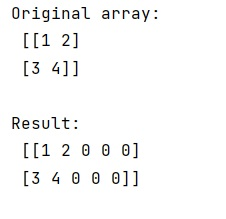# Good ways to 'expand' a NumPy ndarray

Learn what is the good ways to expand a numpy ndarray in Python?
Submitted by Pranit Sharma, on February 11, 2023

NumPy is an abbreviated form of Numerical Python. It is used for different types of scientific operations in python. Numpy is a vast library in python which is used for almost every kind of scientific or mathematical operation. It is itself an array which is a collection of various methods and functions for processing the arrays.

## Expanding NumPy ndarray

Suppose, we are given a 2-dimensional numpy array and we need to expand this numpy array in such a way that it contains some more columns that contain 0 or any other specific number.

For this purpose, we can simply use numpy.pad() method. It takes an argument called pad_width.

These are the number of values padded to the edges of each axis.

• ((before_1, after_1), ... (before_N, after_N)) unique pad widths for each axis.
• (before, after) or ((before, after),) yields the same before and after pad for each axis.
• (pad,) or int is a shortcut for before = after = pad width for all axes.

Let us understand with the help of an example,

## Python code to expand a numpy ndarray

```# Import numpy
import numpy as np

# Creating a numpy array
arr =  np.array([[1,2],[3,4]])

# Display original array
print("Original array:\n",arr,"\n")

# Padding the array with zeroes
res = np.pad(arr, ((0,0),(0,3)), 'constant', constant_values=(0))

# Display Result
print("Result:\n",res,"\n")
```

Output: